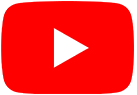# Interactive pages for Algebraic Topology

 Various surfacesA cube with holesTrefoil knotFolding a square to make a torusThe torus as a quotient of the planeLetters of the alphabetLetters grouped by typeCage with two or three holesSimplicesBarycentric coordinatesDifferent triangulations of the sphereThe triangle and the squareSkeleta of simplicesOpen setsFinite element modelA cylinder is homeomorphic to an annulusStereographic projection$\mathbb{R}P^1$ is homeomorphic to $S^1$Gluing two discs to make a sphereLoops on the sphereLoops on the torusA homotopy of the trefoilGeometry of the Möbius stripThe Möbius strip and the circleThe punctured planeThe punctured sphereThe punctured torusWrapping an annulusThe exponential mapThe exponential map is a coveringPath liftingHomotopy liftingSubdivision of a prism $[0,1]\times\Delta_2$ Boundary of a tetrahedronBarycentric subdivision of a tetrahedronThe map $\mu\colon|K'|\to|K|$